# 19 Graph Algorithms You Can Use Right Now

by
Memgraph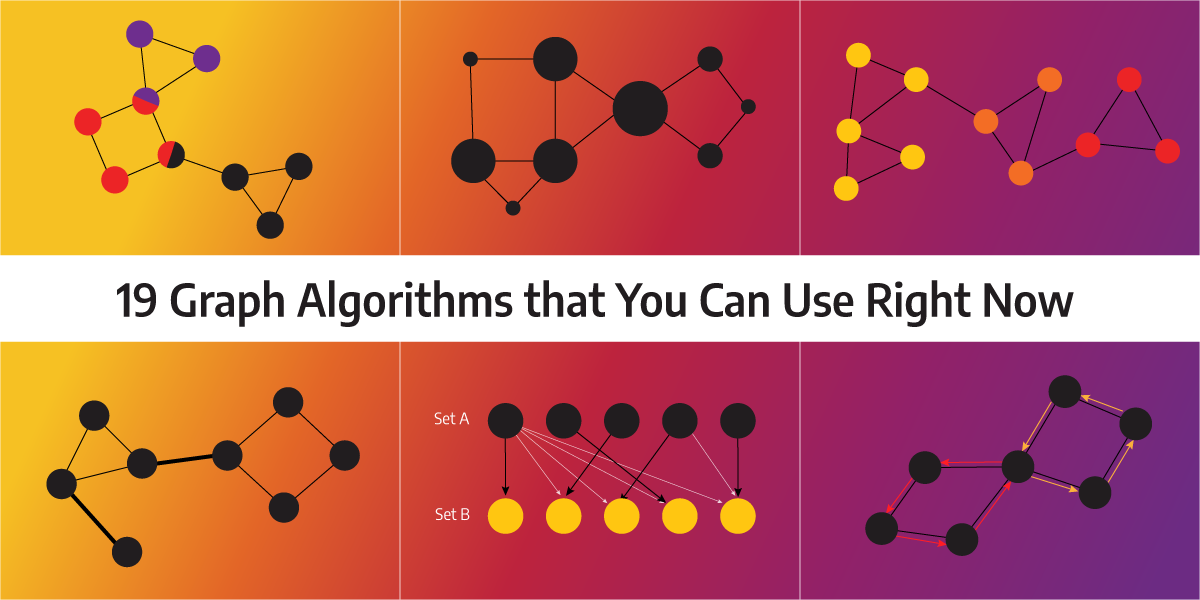The fastest to run any graph algorithm on your data is by using Memgraph and MAGE. It’s super easy. Download Memgraph, import your data, pick one of the most popular graph algorithms, and start crunching the numbers.

Memgraph is an in-memory graph database. You can use it to traverse networks and run sophisticated graph algorithms out-of-the-box.

MAGE is an open-source repository tool supported by Memgraph. MAGE carries different modules and graph algorithms in the form of query modules.

You can choose from 19 graph algorithms along with their GitHub repositories for your query modules. You can use these algorithms with Memgraph and Mage immediately.

## Algorithms List

Here is the list of 19 algorithms that we support. You can use these algorithms immediately with Memgraph (graph DB) and Mage (graph library).

### 1. Betweenness Centrality

Centrality analysis provides information about the node’s importance for an information flow or connectivity of the network. Betweenness centrality is one of the most used centrality metrics. Betweenness centrality measures the extent to which a node lies on paths between other nodes in the graph. Thus, nodes with high betweenness may have considerable influence within a network under their control over information passing between others. The calculation of betweenness centrality is not standardized, and there are many ways to solve it.

It is defined as the number of shortest paths in the graph that passes through the node divided by the total number of shortest paths. The implemented algorithm is described in the paper “A Faster Algorithm for Betweenness Centrality” by Ulrik Brandes of the University of Konstanz.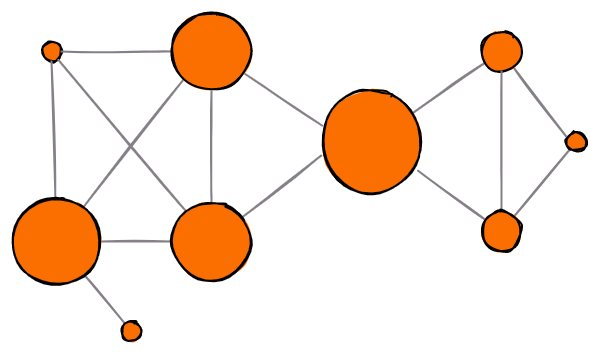A larger circle means larger betweenness centrality. The middle one has the largest amount of shortest paths flowing through it.

### 2. Biconnected Components

Biconnected components are parts of the graph important in the initial analysis. Finding biconnected components means finding a maximal biconnected subgraph. Subgraph is biconnected if:

• It is possible to go from each node to another within a biconnected subgraph
• The first scenario remains true even after removing any vertex in the subgraph

The problem was solved by John Hopcroft and Robert Tarjan with linear time complexity. Depending on the use case, biconnected components may help to discover hidden structures within the graph.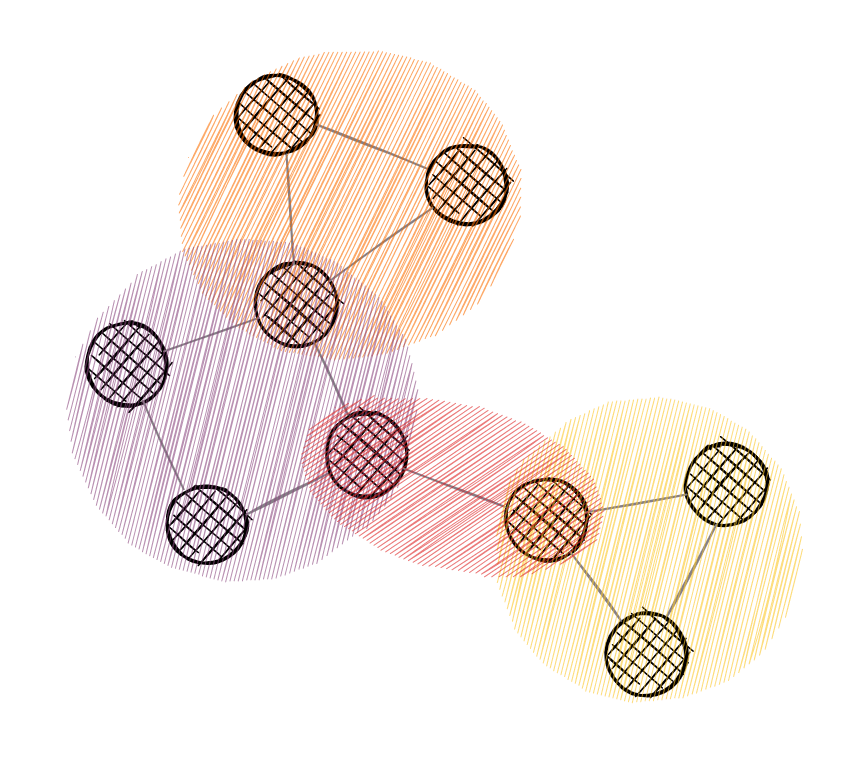Different colors are different components. Multi-colored vertices are articulation points.

### 3. Bipartite Matching

A bipartite graph is a graph in which we can divide vertices into two independent sets, such that every edge connects vertices between these sets. No connection can be established within the set.

Matching in bipartite graphs (bipartite matching) is described as a set of edges that are picked in a way to not share an endpoint. Furthermore, maximum matching is such matching of maximum cardinality of the chosen edge set. The algorithm runs in O(|V|*|E|) time, where V represents a set of nodes and E represents a set of edges.

This little tool can become extremely handful when calculating assignments between entities. Assigning stuff between two sets of entities is done in a large number of industries, and therefore having a method to find it can make the developing process easier.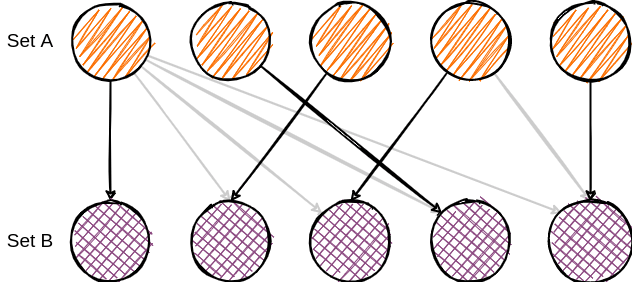### 4. Bridge Detection

As in the real world, the definition of a bridge in graph theory denotes something that divides an entity into multiple components. Thus, more precisely, the bridge in graph theory denotes an edge that, when removed, divides the graph into two separate components.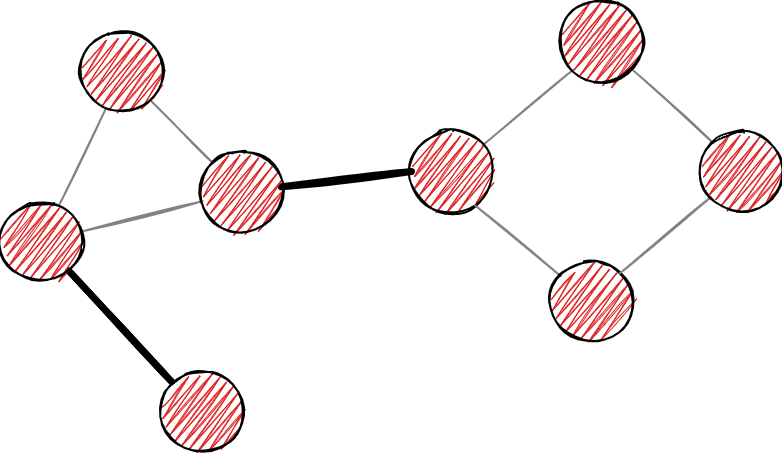Example of bridges on the graph. Bold edges represent bridges.

### 5. Community Detection

The notion of community in a graph represents similarly to what it represents in the real world. Different social circles are examples of such communities. Analogously, in graphs, community represents a partition of a graph, ie a set of nodes. M. Girvan and M. E. J. Newman argue that nodes are more strongly connected within a community, i.e. there are more edges, while on the other hand, nodes are sparsely connected between communities themselves.

There are more potential candidates to address community detection. Among the more popular algorithms are:

1. Louvain community detection - based on modularity optimization - measures network connectivity within a community
2. Leiden community detection - adjustment of Louvain’s algorithm that introduces one level of refinement and brings together strongly connected communities
3. Label propagation - a machine learning technique that assigns labels to unmarked nodes and modifies them with respect to neighbors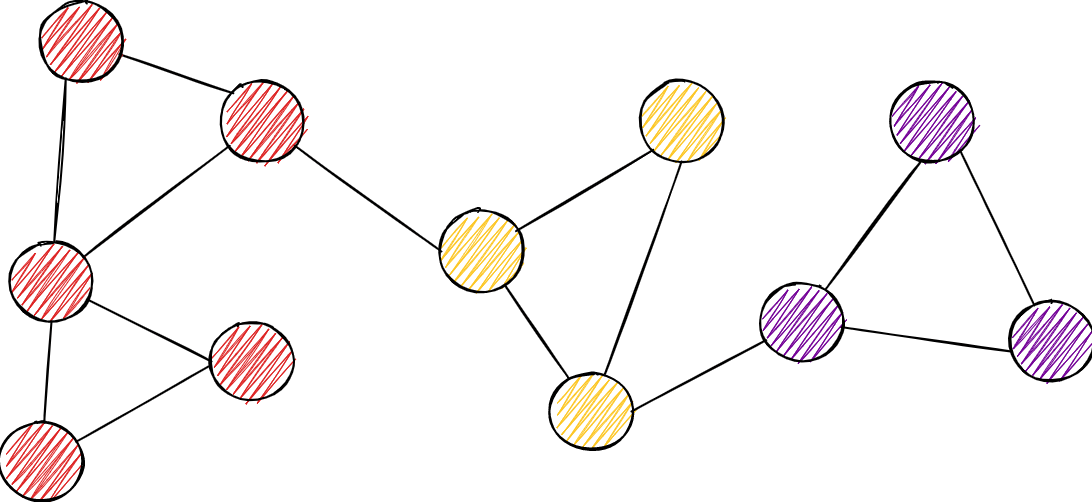Community detection labels each node with a community label. Here, labels are colored in different colors.

### 6. Cycle Detection

In graph theory, a cycle represents a path within the graph where only starting and ending nodes are similar. Furthermore, cycles can be double-connected links between neighboring nodes or self-loops.

The simplest concept of a solution for finding a cycle was defined by Robert W. Floyd in his tortoise and hare algorithm, where a hare moves at twice the speed of a tortoise and thus encounters it if there is a cycle. There are many implementations of cycle detection, and among them, the fastest is Professor Donald B. Johnson from the Pennsylvania State University - Finding all the elementary circuits of a directed graph.

Cycles are not only popular in graph structures but also play an important role in number theory and cryptography. Nevertheless, graph theory concepts are used in other disciplines, and cycle detection is placed as an extremely important tool in initial graph analysis.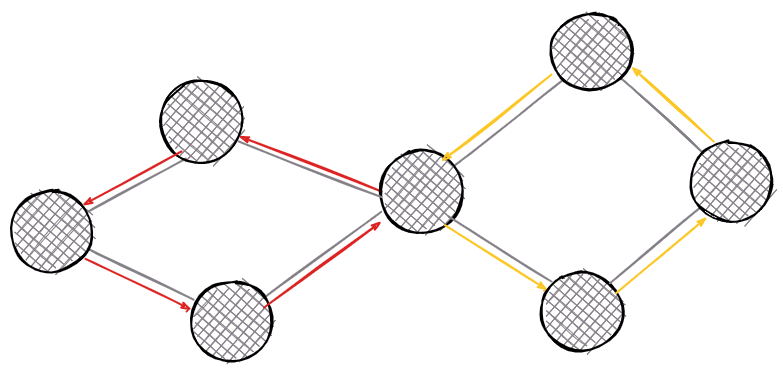There are two cycles in the graph from the examples, each colored differently.

### 7. Graph Coloring

Certain applications require the special labeling of a graph called graph coloring. This “special” labeling refers to the assignment of labels (which we call colors) in such a way that connected neighbors must not be given the same color. Since this problem is NP-hard, there doesn’t exist an algorithm that can solve the problem in a polynomial time. Therefore, various computer science techniques called heuristics are used to solve graph coloring.

Of course, the main part of the problem is in assigning a minimum number of labels. There are greedy algorithms that solve the problem, however not optimal. Using dynamic programming, the fastest algorithm guarantees O(2.44 ^ n) complexity. While on the other hand, there are heuristic applications like the one with genetic algorithms.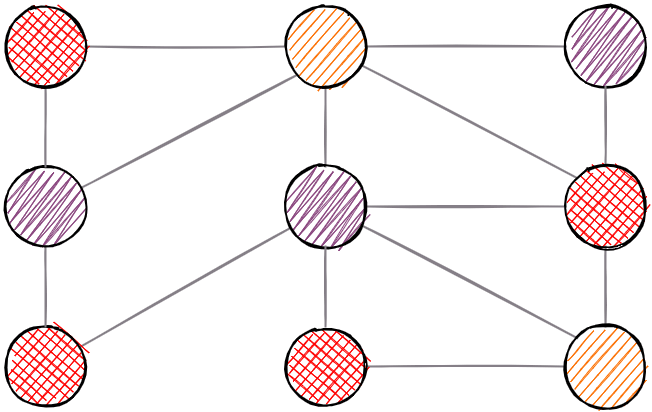Example of graph coloring on a simple graph. Labels are denoted with different colors.

### 8. Node Similarity

The notion of similarity can be described in several different ways, but within graph theory, it encompasses several generally accepted definitions. The similarity of graph nodes is based on a comparison of adjacent nodes or the neighborhood structure. These are traditional definitions that take into account only the layout of the graph. If we extend the definition of a node with additional properties, then it is possible to define comparisons based on these properties as well, but this is not the topic of the methods mentioned here.

The result of this type of algorithm is always a pair of nodes and an assigned value indicating the match measure between them. We will mention only the most popular measures based on neighborhood nodes with their brief explanations.

• Cosine similarity - the cosine of the angle by which the product is defined as the cardinality of the common neighbors of the two nodes, and the denominator is the root of the product of the node degrees
• Jaccard similarity - a frequently used measure in different models of computer science includes the ratio of the number of common neighbors through the total
• Overlap similarity - defined as the ratio of the cross-section of a neighborhood to the less than the number of neighbors of two nodes. Overlap similarity works best in the case of a small number of adjacent nodes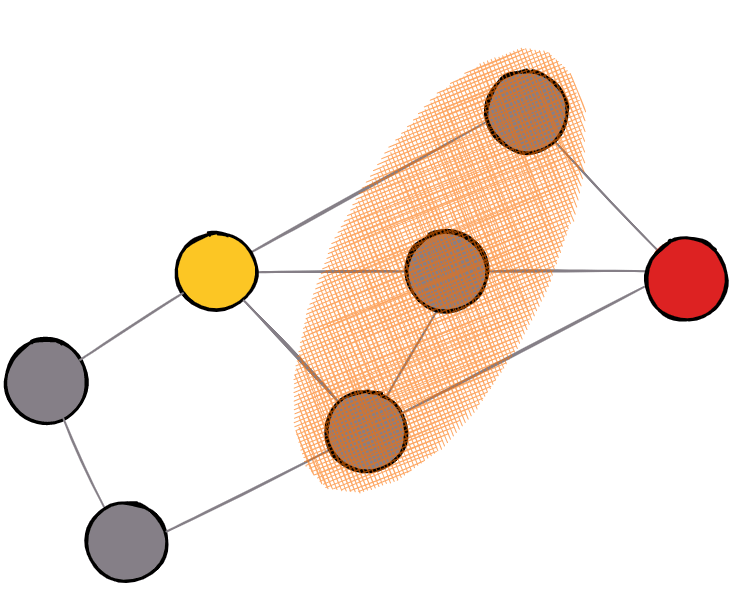Example of a graph where nodes share a neighborhood. This information is used to calculate similarity.

### 9. PageRank

In the domain of centrality measurements, PageRank is arguably the most popular tool. Today, the most popular search engine in the world, Google, owes its popularity solely to this algorithm, developed in the early days by its founders.

If we present nodes as pages and directed edges between them as links, the PageRank algorithm outputs a probability distribution used to represent the likelihood that a person randomly clicking on links will arrive at any particular page.

PageRank can be used as a measure of influence that can be used on a variety of applications, not just on website rankings. A popular type of PageRank is Personalized PageRank, which is extremely useful in recommendation systems.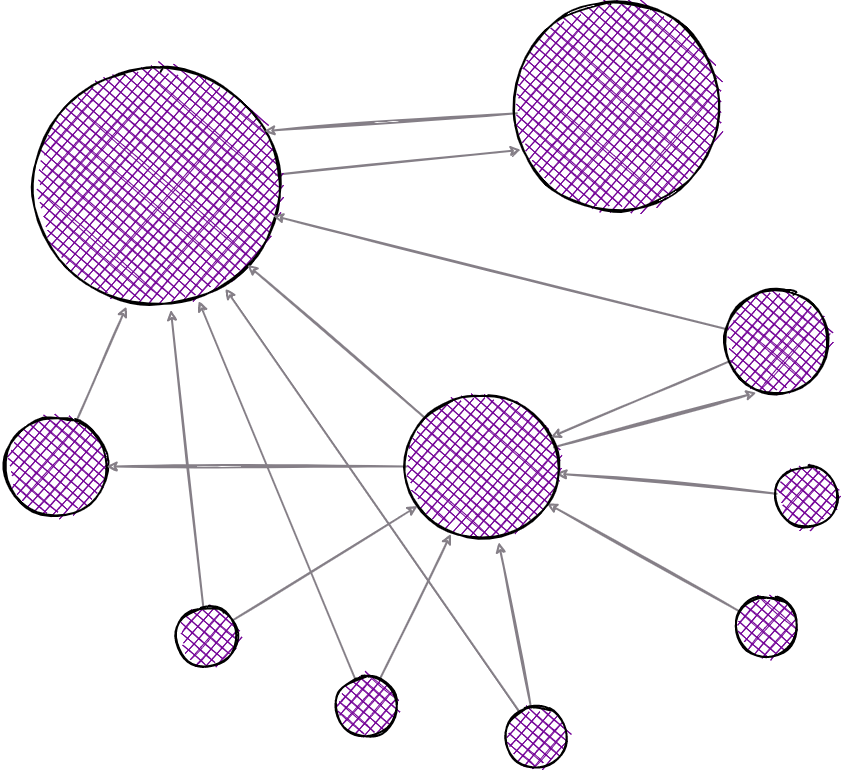PageRank on a simple network. The biggest vertex points to an adjacent vertex and therefore making it more important.

### 10. Union Find

This is yet another important graph analytics algorithm. By using a disjoint-set - a data structure that keeps track of non-overlapping sets, the algorithm enables the user to quickly check whether a pair of given nodes are in the same or different connected components. The benefit of having this structure is that a check on a pair of nodes is effectively executed in O(1) time.

The implementation of the disjoint-set data structure and its operations uses the union by rank and path splitting optimizations described in “Worst-case Analysis of Set Union Algorithms”, developed by Robert Tarjan and Jan van Leeuwen.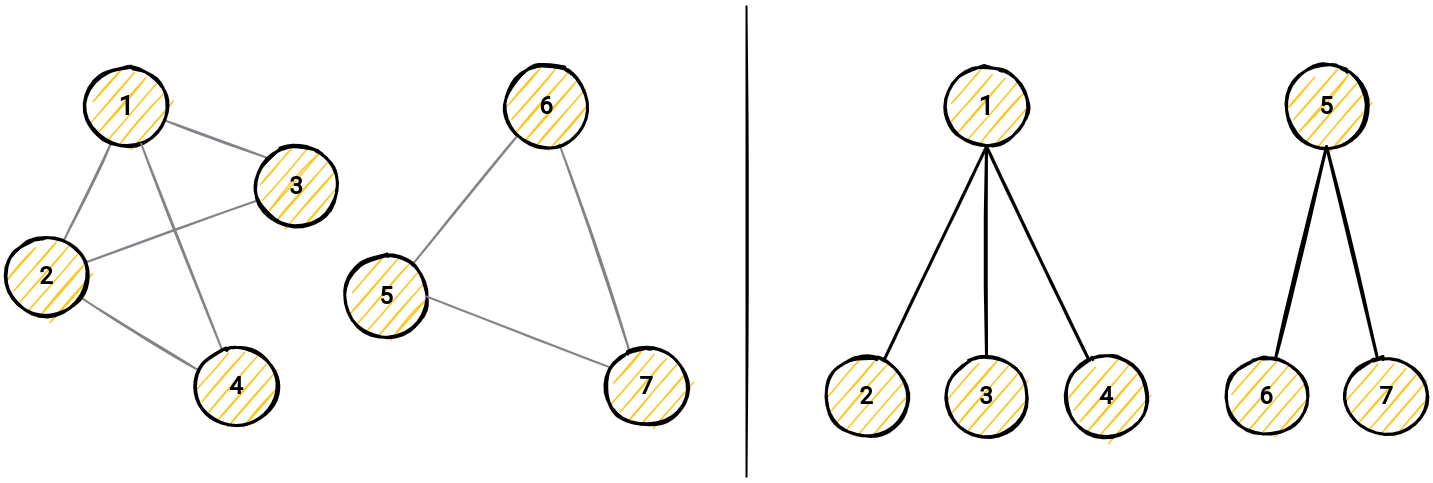Structure of the disjoint set on the right side, and graph example on the left. When checking the components, the algorithm only checks the shallow tree on the left.

### 11. Dynamic node2vec

Dynamic Node2Vec is a random walk-based method that creates embeddings for every new node added to the graph. For every new edge, there is a recalculation of probabilities (weights) that are used in walk sampling. A goal of the method is to enforce that the embedding of node v is similar to the embedding of nodes with the ability to reach node v across edges that appeared one before the other.

Why Dynamic node2vec

Many methods, like node2vec, DeepWalk, focus on computing the embedding for static graphs which has its qualities but also some big drawbacks. Networks in practical applications are dynamic and evolve constantly over time. For example, new links are formed (when people make new friends on social networks) and old links can disappear. Moreover, new nodes can be introduced into the graph (e.g., users can join the social network) and create new links to existing nodes. Naively applying one of the static embedding algorithms leads to unsatisfactory performance due to the following challenges:

• Stability: the embedding of graphs at consecutive time steps can differ substantially even though the graphs do not change much.
• Growing Graphs: All existing approaches assume a fixed number of nodes in learning graph embeddings and thus cannot handle growing graphs.
• Scalability: Learning embeddings independently for each snapshot leads to running time linear in the number of snapshots. As learning a single embedding is computationally expensive, the naive approach does not scale to dynamic networks with many snapshots

Dynamic Node2Vec is created by F.Beres et al in the “Node embeddings in dynamic graphs”.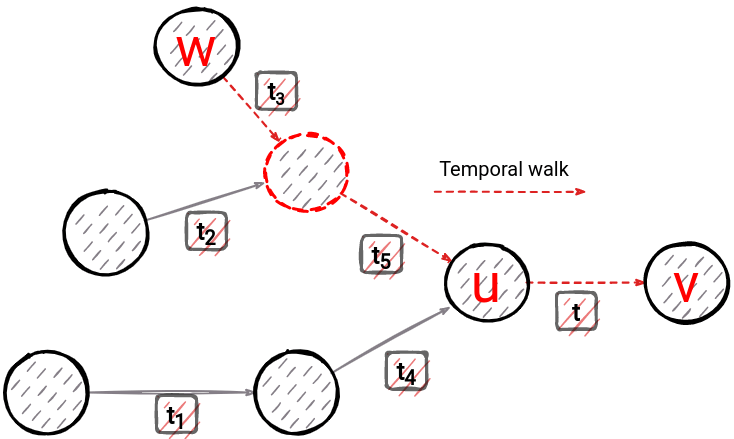### 12. Dynamic PageRank

In the domain of estimating the importance of graph nodes, PageRank is arguably the most popular tool. Today, the most popular search engine in the world, Google, owes its popularity solely to this algorithm, developed in the early days by its founders.

If we present nodes as pages and directed edges between them as links the PageRank algorithm outputs a probability distribution used to represent the likelihood that a person randomly clicking on links will arrive at any particular page.

The need for its dynamic implementation arose at the moment when nodes and edges arrive in a short period of time. A large number of changes would result in either inconsistent information upon arrival or restarting the algorithm over the entire graph each time the graph changes. Since such changes occur frequently, the dynamic implementation allows the previously processed state to be preserved, and new changes are updated in such a way that only the neighborhood of the arriving entity is processed at a constant time. This saves time and allows us to have updated and accurate information about the new values of the PageRank.

There are also some disadvantages of this approach, and that is that such an approach does not guarantee an explicitly correct solution but its approximation. Such a trade-off is common in computer science but allows fast execution and guarantees that at a large scale such an approximation approaches the correct result.

Valuable work explaining how to quickly calculate these values was developed by Bahmani et. al., engineers from Stanford and Twitter. The paper is worth reading at [Fast Incremental and Personalized PageRank](http://snap.stanford.edu/class/cs224w-readings/bahmani10pagerank.pdf 📖).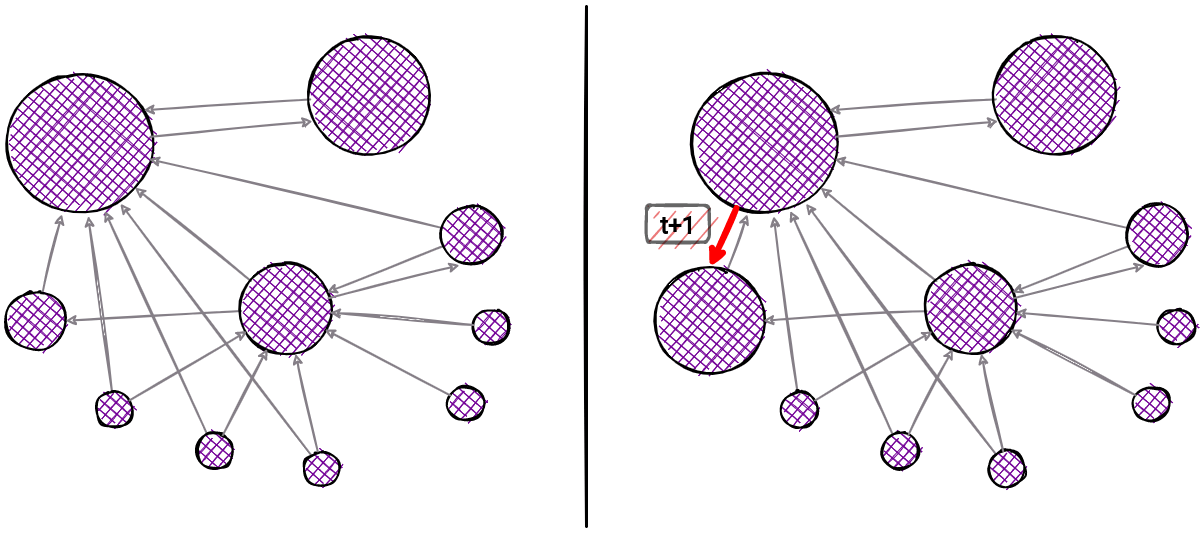### 13. Dynamic Community Detection

To address the hidden relations among the nodes in the graph, especially those not connected directly, community detection can provide help. This familiar graph analytics method is being solved in various different ways. However, demand for scale and speed has increased over the years and therefore led to the construction of dynamic community detection algorithms. To leverage the needs for scalability and speed, community detection lends itself well to dynamic operations for two reasons:

• Complexity: algorithms often have high time complexity that scales with the number of nodes in the network
• Locality: community changes tend to be local in scope after partial updates.

Academic research of temporal graphs yielded LabelRankT: Incremental Community Detection in Dynamic Networks via Label Propagation (Xie et al.).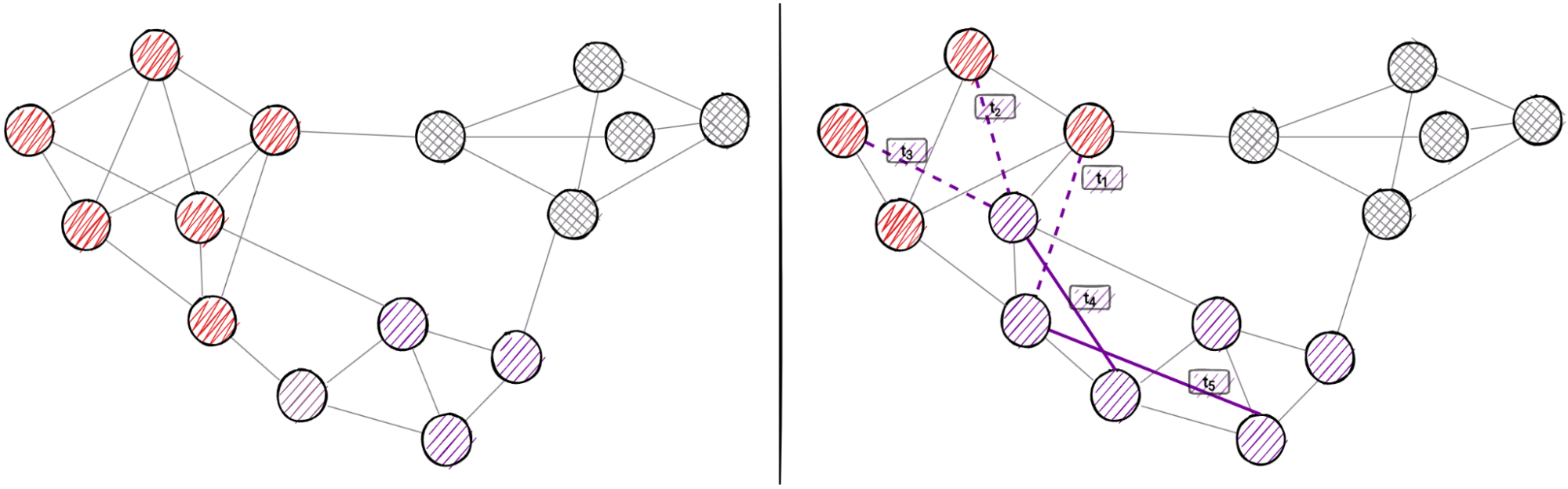Illustration of how a dynamic community detection algorithm adapts to local changes

### 14. Graph Neural Networks (GNN)

Machine learning methods are based on data. Because of everyday encounters with data that are audio, visual, or textual such as images, video, text, and speech - the machine learning methods that study such structures are making tremendous progress today.

Connection-based data can be displayed as graphs. Such structures are much more complex than images and text due to multiple levels of connectivity in the structure itself which is completely irregular and unpredictable. With the development of neural networks organized in the structure of graphs, the field of graph machine learning is improving.

Applying the same principle used, for example, in images (convolutions) to graphs would be a mistake. Such principles are based on grid structures, while on graphs of arbitrary sizes, complex topologies, and random connections applying the same strategy would result in a disaster.

All convolutional network graph methods are based on message propagation. Such messages carry information through a network composed of nodes and edges of the graph, while each node entity carries its computational unit. The task of each node is to process the information and pass it on to the neighbors.

Various possibilities have been developed that enable machine learning with graph neural networks. Starting with the graph of convolutional networks published in “Spectral Networks and Deep Locally Connected Networks on Graphs” (Bruna et al, 2014).

Today’s most popular models are GraphSAGE, Graph Convolutional Networks (GCN), Graph Attention Networks (GAT), and Temporal Graph Networks (TGN) - important for dynamic networks.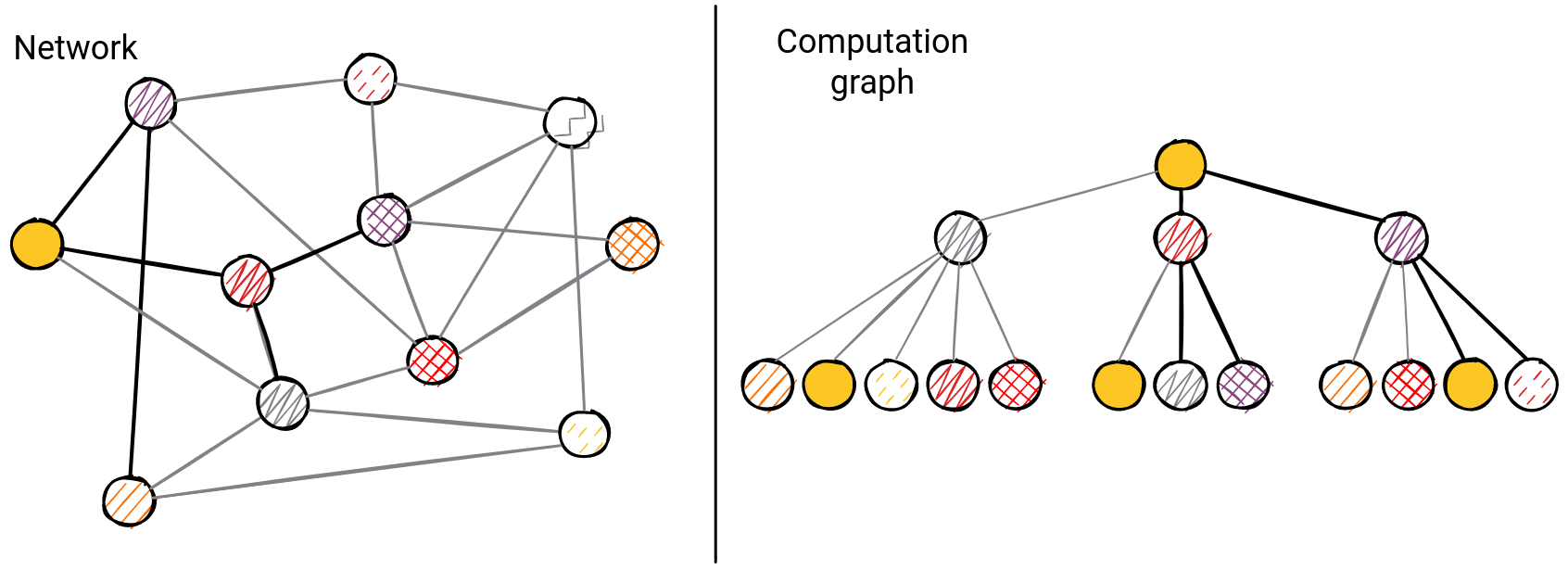The above network shows the computation graph of message propagation in GNNs.

### 15. Graph Classification

Let’s look at one useful tool that allows you to analyze a graph as a whole. Graph classification enables this. The structure and arrangement of nodes can reveal some hidden features in a graph.

So, for example, fraudsters who have a common pattern of behavior can be detected by searching that pattern in the graph of their connections.

To make this possible, the main technique is to design features over the structure of the graph itself and then apply a classification algorithm. This can be achieved in several ways:

• Graphlet features - the number of a particular graphlet indicates a single element of the feature vector
• Weisfeiler-Lehman kernel - color refinement, teaching colors to the neighborhood until convergence is achieved
• Graph Deep Learning - designing a network that can extract a deeper feature depending on the structure of the graph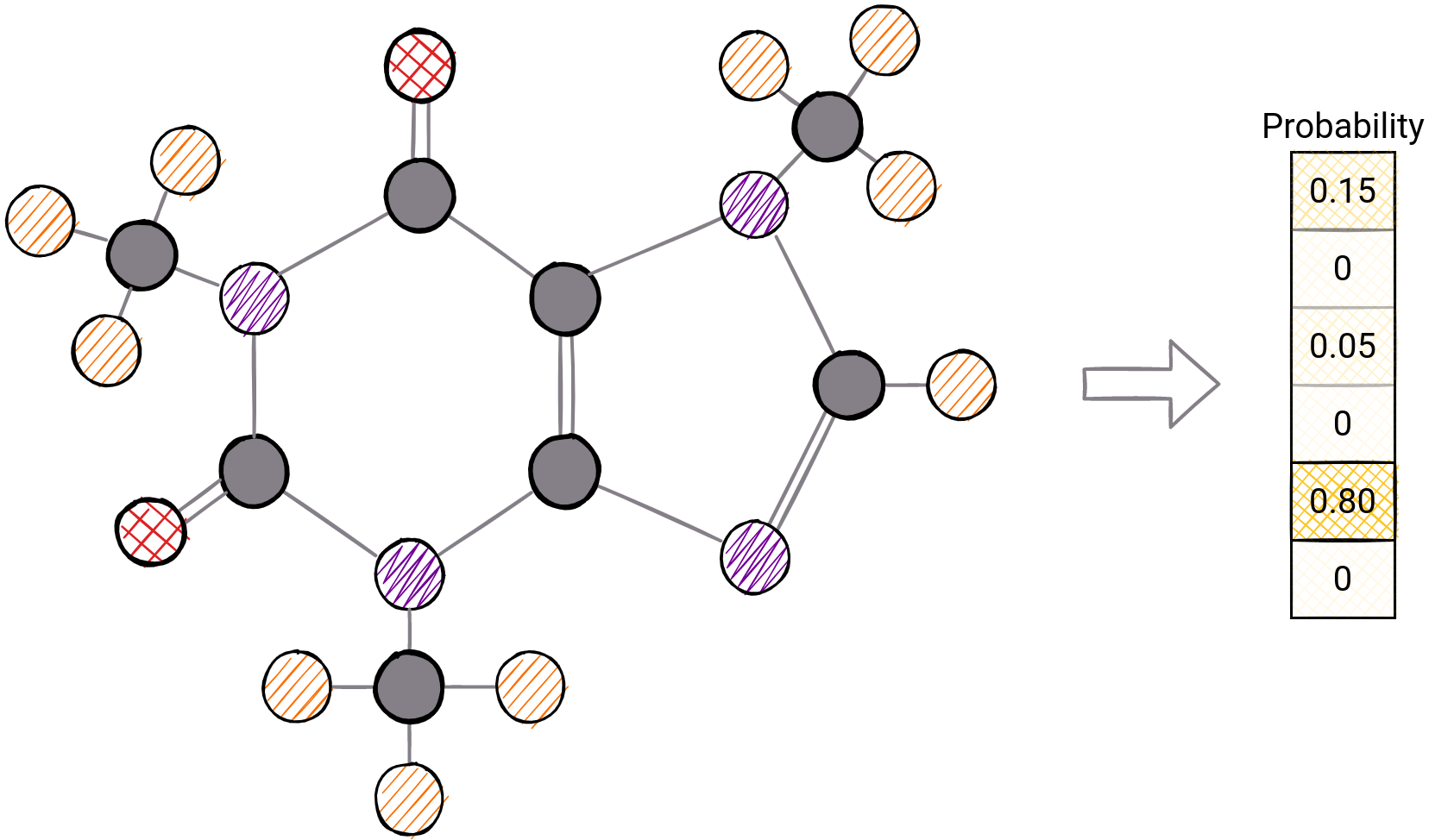Probability of different labels on graph classification for molecular structures

Link prediction is the process of predicting the probability of connecting the two nodes that were not previously connected in a graph. A wide range of different solutions can be applied to such a problem.

The problem is of great importance in recommendation systems, co-authorship prediction, drug discovery, and many, many more.

Solving methods range from traditional to machine learning-based. Traditional methods are mostly based either on neighborhood similarity. A link between two nodes is more likely to exist if there are many common neighbors. These are:

On the other hand, such a prediction can be learned from the node endpoints by looking at similarity metrics. The more similar the nodes are, the greater the likelihood of connectivity between them. Cosine similarity and Euclidean distance are one example of such.

Then, there are the most advanced models so far and they are based on graph embeddings, which serve as features for further classification tasks. Likewise, it is possible to drive graph neural network (GNN) methods for the same task.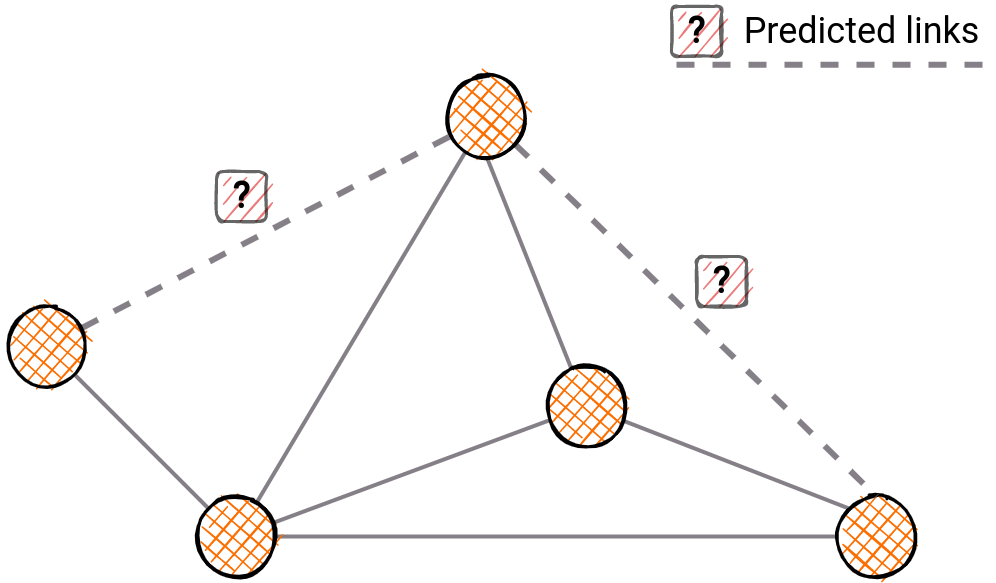Predicted relationships within the certain community

### 17. Node Classification

Prediction can be done at the node level. The basis of such prediction systems are features extracted from graph entities.

Extracting a feature can be a complicated problem, and it can be based on different graph attributes — node properties, node adjacency, or the structure of the neighborhood.

Traditional methods of extracting knowledge from nodes include measures of centrality, importance, or feature structure such as graphlets.

Somewhat more advanced methods are extracting the embedding of individual nodes, and then a prediction algorithm that takes knowledge from the embeddings themselves. The most popular such tool is Node2Vec.

However, these methods are only a few. Today’s graph machine learning is being developed and among them, we distinguish many different models such as:

and many, many more. This task has become quite popular and is used in many industries where knowledge is stored in the form of a graph structure.

Previously labeled nodes can be used to determine the class of unclassified ones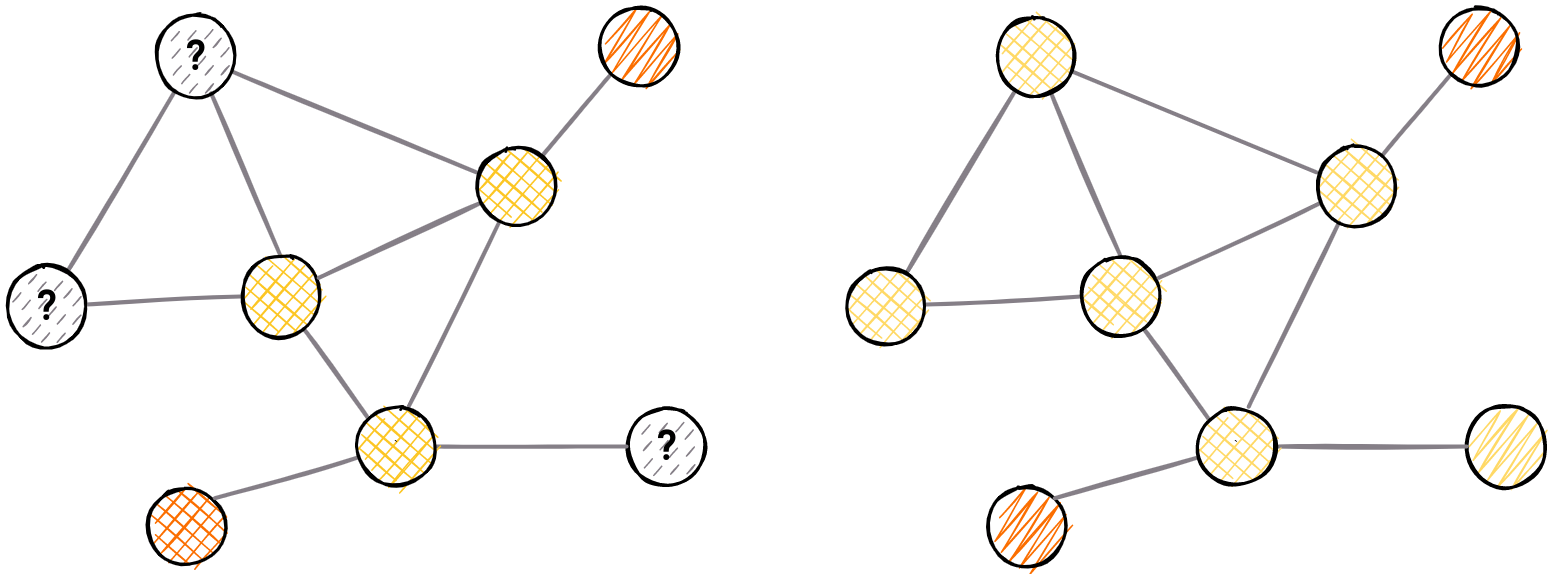### 18. Node2Vec

Embedding methods serve to map graph entities into n-dimensional vectors. The goal of such an approach is to map closely related entities to vectors with a high degree of similarity according to the chosen method of similarity estimation.

Node2Vec stands out as the most popular method. It is based on random walks. The point of this method is mapping nodes that are most likely to be within a common random walk to the same place in n-dimensional space. The method was developed by Aditya Grover and Jure Leskovec, professors at Stanford University in their paper “node2vec: Scalable Feature Learning for Networks

The optimization of the mapped vectors themselves is done by a well-known machine learning method such as gradient descent. In the end, the result obtained can be used for node classification or link prediction, both truly popular.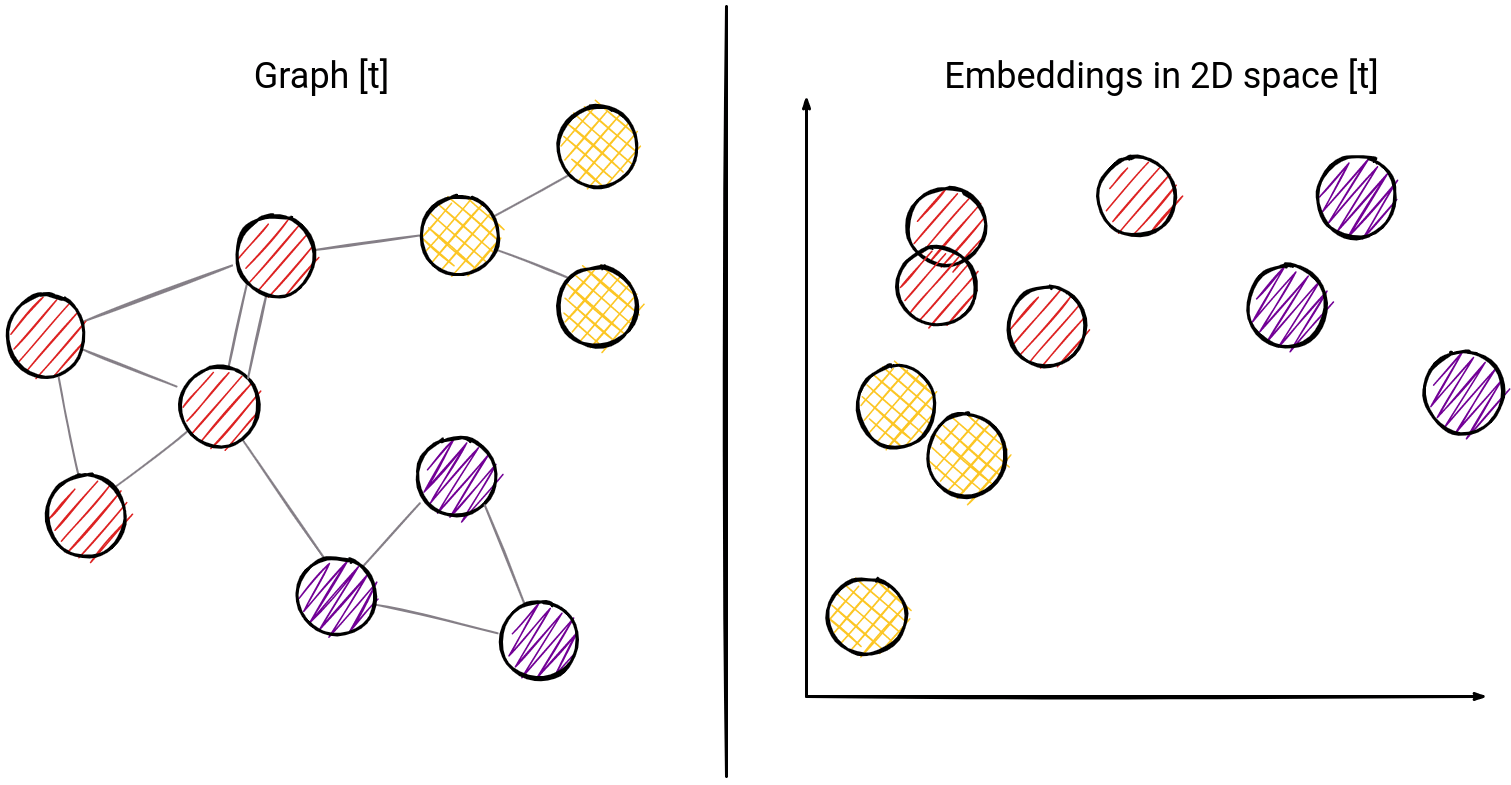Illustration of how graph embeddings can be mapped to 2D space. Boundaries between classes are more visible than in a graph.

### 19. Graph Clustering

In graph theory, graph clustering is used to find subsets of similar nodes and group them together. It is part of graph analysis which has been attracting increasing attention in recent years due to the ubiquity of networks in the real world.

Graph clustering also known as network partitioning can be of two types:

• structure-based
• attribute-based clustering

The structure-based can be further divided into two categories, namely community-based, and structurally equivalent clustering.

Community-based methods aim to find dense subgraphs with a high number of intra-cluster edges and a low number of inter-cluster edges. Examples are the following algorithms:

Structural equivalence clustering, on the contrary, is designed to identify nodes with similar roles (like bridges and hubs). An example is SCAN: A Structural Clustering Algorithm for Networks

One example that can vary between community-based and structurally equivalent clustering is Node2Vec.

Attribute-based methods utilize node labels, in addition to observed links, to cluster nodes like the following algorithm: Graph clustering based on structural/attribute similarities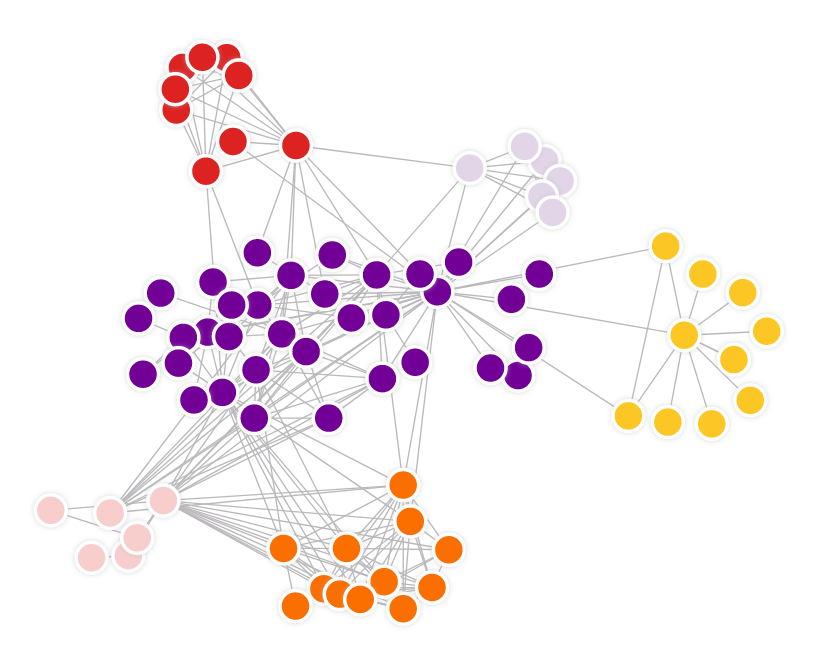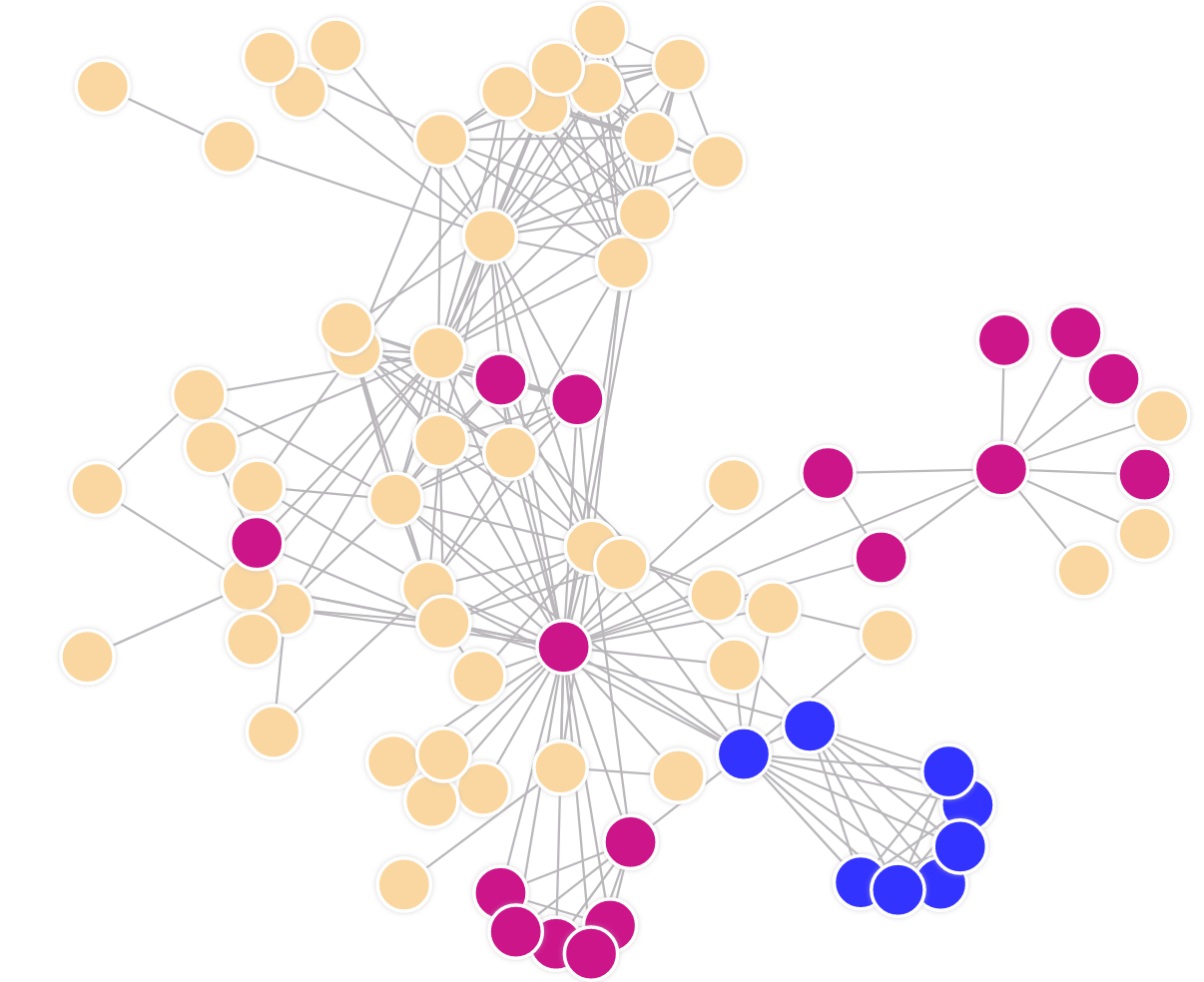Structure-based community clustering

## Conclusion

These are the top 19 graph algorithms to check out - there’s everything in here from PageRank to Centrality. Give them a go and let us know if you have any challenges in using them with Memgraph by posting on Discourse or Discord

pagerank-algorithm-for-graph-databases
Graph Algorithms
PageRank
PageRank Algorithm for Graph Databases

What is PageRank algorithm? How can it be used in various graph database use cases? How to use it in Memgraph? If these questions are keeping you up at night, here is a blog post that will finally put your mind at ease.

by
Vlasta Pavicic
January 27, 2023
become-an-inspector-for-a-day-and-detect-fraudsters-with-graph-ml-on-memgraph
Machine Learning
Fraud Detection
Use Cases
Graph Algorithms
Become an Inspector for a Day and Detect Fraudsters With Graph ML on Memgraph!

This blog post deals with solving fraud detection problems with graph machine learning. Learn how to load data, training and plot to find out who did it! It’s elementary, my dear reader.

by
Mateo Dujic
December 7, 2022
building-a-recommendation-system-for-telecommunication-packages-using-graph-neural-networks
Graph Algorithms
Machine Learning
Recommendation Engine
Use Cases
A Hyperparametrization Is All You Need - Building a Recommendation System for Telecommunication Packages Using Graph Neural Networks

Graph Neural Networks can be used for a variety of applications but do you know what it takes to create a great recommendation system? Dive deep into the math of GNNs, implement a link prediction module and show everyone how stunning graph machine learning can be!

by
Andi Skrgat
December 5, 2022